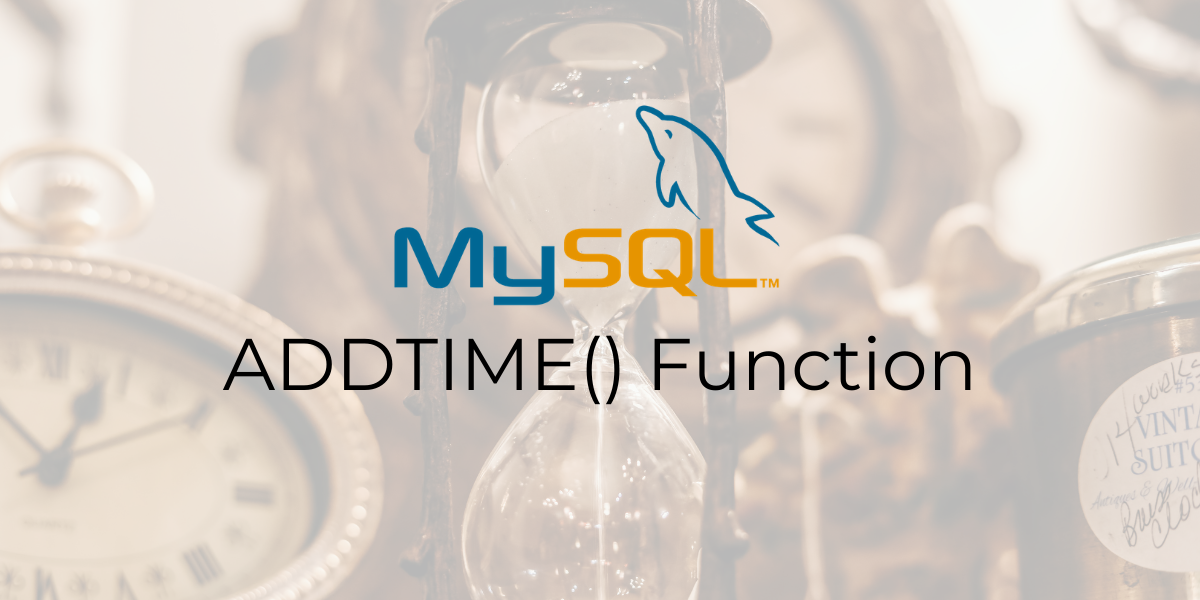In this tutorial, we will study the MySQL `ADDTIME()` function. Suppose you have a table which contains the names of shops and their opening time. Now suppose during winters, these shops decide to open an hour late. You have been tasked with adding an hour to all the time values.

This situation calls for the use of MySQL `ADDTIME()` function. The MySQL `ADDTIME()` function is used to add a time interval to a time or datetime value. It takes in two arguments – the first one is the original time/DateTime value and the second is the time interval to be added. Let us take a look at the syntax and examples of the MySQL `ADDTIME()` function.

Recommended read – MySQL TIMEDIFF() and MySQL DATEDIFF()

```ADDTIME(time, add_time_val)
```

Where ‘time’ is the time/datetime value to be modified and,

‘add_time_val’ is the time interval to add to ‘time’. This value can be positive or negative.

Let us kick things off with some basic examples. Let us write queries for the following to problems –

• Adding 10 minutes and 11 seconds to 1 minute.
• Adding 2 hours and 15 seconds to 2 hours and 15 seconds.

We will use the SELECT statement and an alias called ‘Result’ to make our output readable. The queries are –

```SELECT ADDTIME('00:01:00','00:10:11') AS Result;
```

And the output is –

### Limitation of the Time Data Type

One thing you should know while working with the time data type is that it has a major limitation. The time data type is limited to a range from -838:59:59 to 838:59:59. This means it does not recognize values out of that range. Let us see an example where the addition of two time values results in a value outside the above range. The query is –

```SELECT ADDTIME('500:00:15','500:00:56') AS Result;
```

And the output is –

As you can make out, this value is incorrect. This is because `ADDTIME()` stops counting after it reaches the time value ‘838:59:59.’ Any result that will have a value greater than ‘838:59:59’ will return ‘838:59:59’ regardless.

### MySQL ADDTIME() With Fractional Seconds Precision

We can add time values with fractional seconds precision using the `ADDTIME()` function. Let us see a couple of examples of the same. Consider the below queries.

```SELECT ADDTIME('02:00:30.1255','03:30:00.148697') AS Result;
```

And the output is –

### MySQL ADDTIME() With Datetime Values

We can also increment datetime values with `ADDTIME()`. Let us see an example of this.

```SELECT ADDTIME('2021-06-01 00:01:10', '15 06:30:00') AS Result;
```

In the above query, we have a datetime value – ‘2021-06-01 00:01:10.’ The ‘add_time_val’ parameter has the value – ’15 06:30:00’. The 15 in that value stands for 15 days while 06:30:00 is the time value. The above query will add 15 days, 6 hours and 30 minutes to the give datetime value. The output is –

Let us see another example. Consider the below query.

```SELECT ADDTIME('2021-06-01 00:01:10', '25:30:00') AS Result;
```

Now notice that we are adding 25 hours and 30 minutes to the given datetime value. `ADDTIME()` is smart. Since the time value is greater than 24 hours, it increments the date value as well. We can see that in the below output.

We can include functions like `CURTIME()` that return a time value inside the `ADDTIME()` function. Let us write a query that adds two hours to the current time.

```SELECT CURTIME(), ADDTIME(CURTIME(), '2:00:00') AS 'Plus 2 Hours';
```

And the output is –

### ADDTIME() With Wrong Time Values

`ADDTIME()` returns NULL if we pass incorrect time values to it. An incorrect time value is one in which the minute or the second value is greater than 59. Let us see an example of this.

```SELECT ADDTIME('02:00:15','20:70:15') AS Result;
```

And the output is –

### Working with Tables

Consider the below Shops table.

Let us get back to the problem I mentioned in the beginning of the article. Suppose you have a table which contains the names of shops and their opening time. Now suppose during winters, these shops decide to open an hour late. You have been tasked with adding an hour to all the time values. Let us now write a query for this using the `UPDATE` statement and `ADDTIME()` function.

```UPDATE Shops SET OpeningTime=ADDTIME(OpeningTime, '01:00:00');
SELECT * FROM Shops;
```

And the output is –

## Conclusion

The `ADDTIME()` function is very useful. Basic addition operations on time and datetime values are very important and have a plethora of use cases. I would encourage you to practice some more queries with this function.